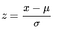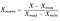# Standardization and normalization — An approach to achieve a better performing ML model

- A quick guide on feature scalingImage Source: Mitya Ivanov via Unsplash

Often in machine learning, we come across an imbalanced, non-normal, and skewed dataset. What does that mean and how does that impact our machine learning model?

In this article, we will cover the impact of imbalanced and skewed data on the ML model and ways to handle this data during the data preprocessing phase in order to enhance the performance of our model. Before we dive into the ways to deal with such datasets, let’s explore data preprocessing and what imbalanced and non-normal data means.

# What is Data Preprocessing?

It is the feature engineering part of machine learning where the real-world data is engineered or transformed into a machine-understandable format. Raw data or real-world data if sent to the model without processing might result in errors and false results as no raw data is fully fit for the direct model training phase. Data preprocessing reduces the complexity in data and brings out only those features which are necessary for model training, thereby simplifying the relationship between features. It is the most important concept in machine learning and contributes significantly to the performance of the model.

An imbalanced dataset is majorly a concept of supervised learning classification in which the data points available for different classes vary in huge numbers. For ease, in the case of binary classification balanced data would mean 50% of data available for both classes.

A skewed dataset statistically is a distortion in data that makes it skewed either to the left or the right. It is the unsymmetrical nature of this data that makes it non-normal. Imbalanced data indeed is a subset of skewed data only.

# To the rescue . . .

There are techniques that help achieve normal distribution in the data (i.e. bell-shaped curve) during the phase of feature engineering or data preprocessing. Let’s define the most commonly used techniques –

Standardization (Z-score) is a technique used to bring the data to a similar scale. This scaling technique follows the data values to be centered around mean with standard deviation being 1 i.e. the mean of “standard normal variable” becomes 0 and standard deviation becomes 1. Here is the formula for standardization where μ is the mean and σ is the standard deviation from the mean –Standardization Formula

Normalization (Min-Max) is a scaling technique used to bring all elements of the dataset in the range of 0 and 1. This generally applies to numeric features(columns) and brings them to a common scale. Here is the formula for normalization –Normalization Formula

Unit Vector Normalization scales the data to a unit sphere. The transformed data is seen as a bunch of vectors having different directions on the n-dimensional unit sphere when applied to the complete dataset.

# Why scale up your data?

While comparing measurements with different units, the goal of standardization or normalization is to bring variables to a similar scale.

• There is a high chance of bias when variables are with different scales and hence do not contribute equally or fairly to model performance.
• The learning rate of the algorithm is determined by the feature with the largest range when features have different ranges. Therefore, scaling the feature surely speeds up the training time of the algorithm.
• Scaling the data improves the overall accuracy of the model.

Normalization is applied to numeric columns without having to disturb the difference in the range of values. It is required only when features have different ranges and therefore is not required for every dataset.

# Standardization v/s Normalization

Normalization is a preferred technique when you have no idea of the distribution of your data or when it is known that the distribution is not Gaussian (a bell-shaped curve). It is useful when the data is having different scales and the ML algorithm used for training does not make assumptions about the distribution of the data, for example, in the case of k-nearest neighbor and neural networks.

Standardization is a preferred technique when either you know or assume that the data has a Gaussian distribution i.e. a bell-shaped curve. It surely works another way as well, but the technique is more efficient with normal distribution. Standardization is useful when the data is having different scales and the ML algorithm used for training makes assumptions about the distribution of the data to be gaussian, for example, in the case of linear regression and logistic regression.

# Other commonly used Data preprocessing techniques...

As a data scientist, one would know how to play around with different types of data. There are different mining techniques for different nature of data. Let’s try to understand some of these by categorizing them into subsets of preprocessing phase.

1. Data Cleaning –
• Check for null/empty values — Check for empty values in the dataset and then depending upon the weightage of data, you can choose to either remove the null values or fill them logically such as by taking the mean of the data and replacing null values with mean.
• Check for duplicates — Check for duplicates in the dataset and remove them in order not to give a particular (duplicate) data a bias in the machine learning model.
• Outlier removal — outliers should be removed from the data in order to make the data symmetrical.

2. Data Transformation –

• Normalization/Standardization — It is done in order to bring the data values in a specified range of scale. We discussed these techniques at the beginning of this article.
• Feature Encoding — A subset of normalization is encoding the features. Depending upon if the data is categorical (nominal or cardinal) or numeric, there can be different encoding techniques. The most commonly used categorical encoding techniques are one-hot encoding and label encoding.

3. Data Reduction –

• Aggregate the data — This is done in order to take the aggregated values to put the data in a better perspective.
• Correlation matrix — Plot a correlation matrix and remove the features which are highly positively correlated as they might serve similarly in training the model.
• SMOTE (Synthetic Minority Oversampling Technique) — It is one of the best techniques to solve an imbalanced dataset problem. It is used to balance class distribution by randomly increasing minority class examples by replicating them.
• NearMiss (Under-sampling) — It works opposite to the over-sampling technique. It is used to balance class distribution by randomly removing majority class examples.
• PCA (Principal Component Analysis) — This is the most commonly used technique for dimensionality reduction (reduction of features). It solves the “Curse of dimensionality” (the problem of data analysis becomes significantly harder as the dimensionality of the data increases) and overfitting problem.

# Conclusion

We have learned various ways to handle imbalanced and skewed data while the data preprocessing phase. Because of the reduced complexity in data and the simplified relationship between data features, processed data can give unexpected results with increased accuracy of the model. Therefore, while dealing with data in machine learning, it is mandatory to undergo data mining so as to make it fit for model training and learning.

# References

https://www.geeksforgeeks.org/data-preprocessing-in-data-mining/

https://towardsdatascience.com/data-pre-processing-techniques-you-should-know-8954662716d6

https://medium.com/@swethalakshmanan14/how-when-and-why-should-you-normalize-standardize-rescale-your-data-3f083def38ff

https://www.analyticsvidhya.com/blog/2020/04/feature-scaling-machine-learning-normalization-standardization/

https://towardsdatascience.com/data-preprocessing-concepts-fa946d11c825

Data Scientist || MLOps Engineer || Co-author of book “Applications of Reinforcement Learning to Real-World Data (2021)” || AI Tutor & Mentor

## More from Kajal Singh

Data Scientist || MLOps Engineer || Co-author of book “Applications of Reinforcement Learning to Real-World Data (2021)” || AI Tutor & Mentor